Osmotic energy

This post’s subject is about osmotic energy. It is one more alternative of renewable and clean energy.

When the fresh water enters in contact with sea water, the salt diffuses until both has the same concentration, or the equilibrium. The concentration gradient is the difference of solute density (salt ions) in two solvent (water) regions.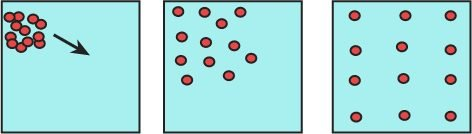The gradient concentration of ions can be used to energy generation. Exist two types of osmotic energy generation: pressure retarded osmosis (PRO) and reverse electrodialysis (RED).

Pressure retarded osmosis (PRO)

If sea water and fresh water are separated by a semipermeable membrane, this membrane allows the passage of water but not ions. The liquid with bigger quantity of salt will have an osmotic pressure.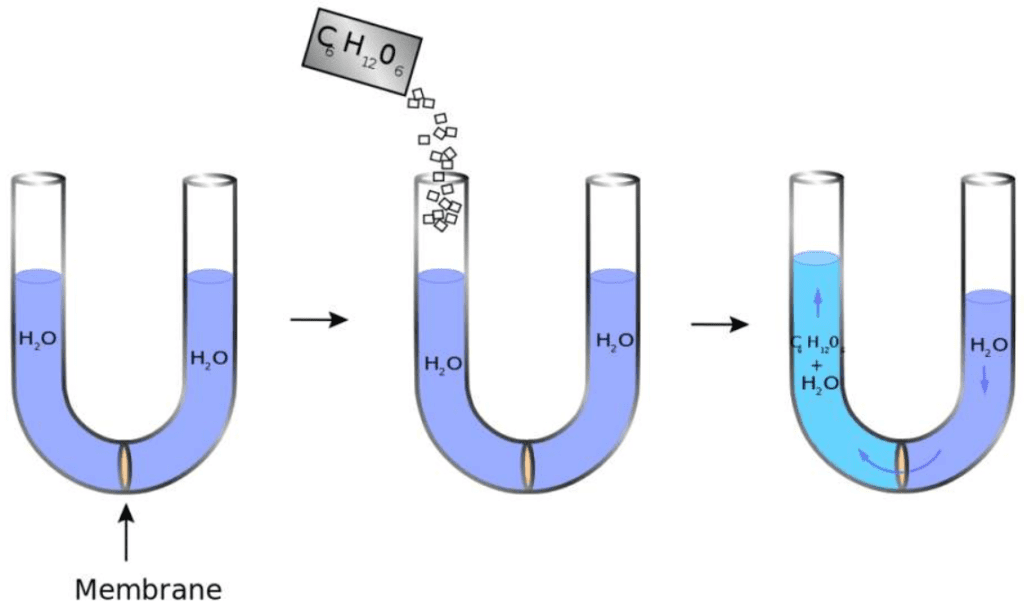The osmotic pressure can be used to turn a turbine and produce electric energy. Waters pass through filters and pumps before reach containers with membrane.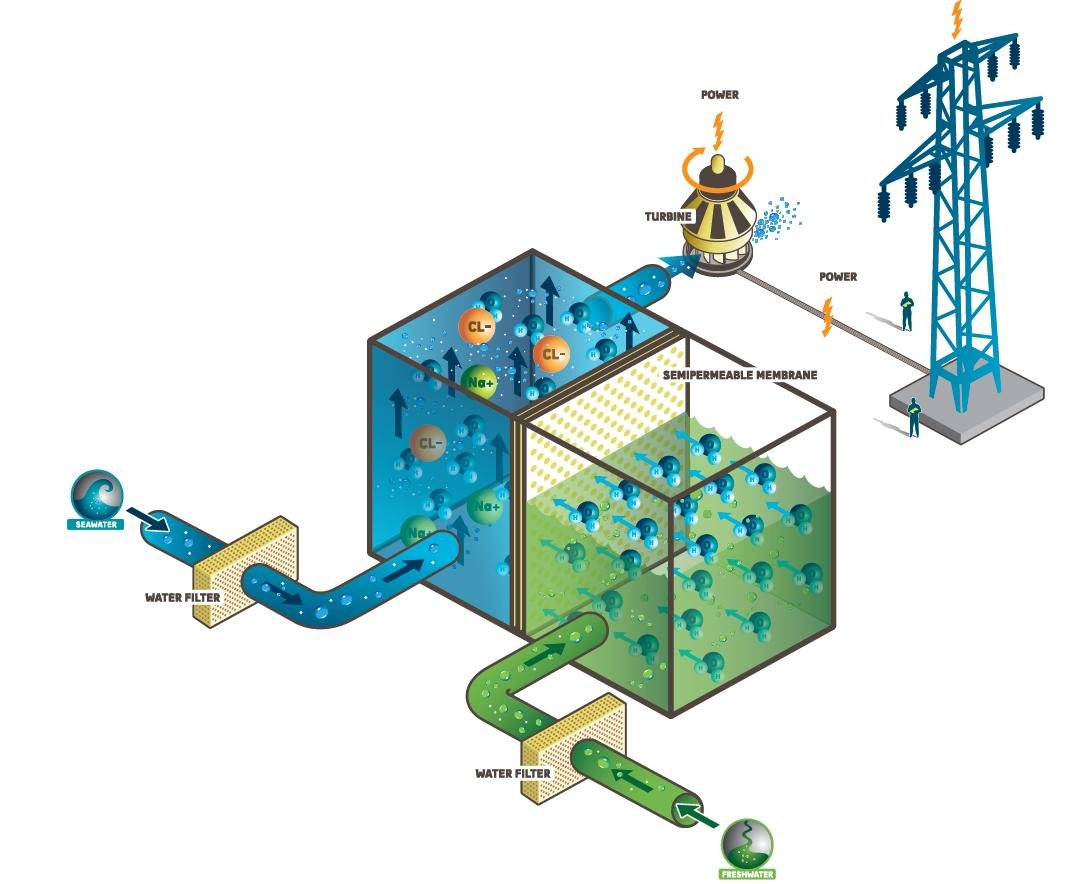When bigger the salt concentration, bigger the osmotic pressure and energy generation. Below are the materials which can be used to semipermeable membrane.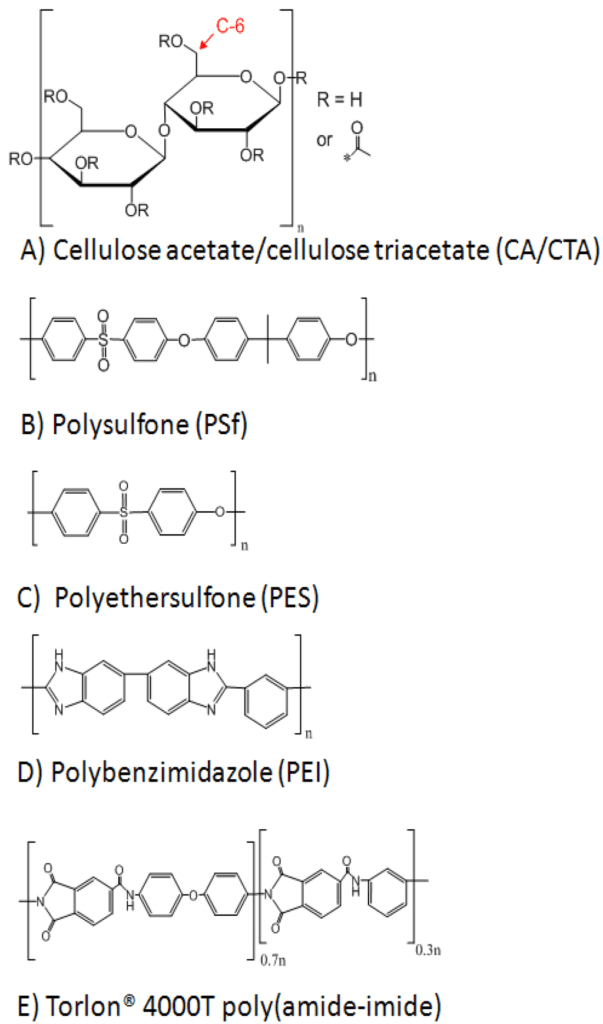Source: (Alsvik e Hägg, 2013)

This is the formula of osmotic pressure $\pi$.

$\pi =\frac{n}{V_{m}}iRT$

• $n$ is the number of solute particles in mols;
• $V_{m}$ is the solvent volume in liters;
• $R$ is the gas constant, whose valor is 0,0821 $\frac{L\cdot atm}{mol \cdot K}$;
• $T$ is the temperature in kelvin (K);
• $i$ is the van`t Hoff factor, indicates the number of ions created when a molecule is dissolved in a solvent. For example, sodium chloride ($NaCl$) dissolved in water produce 2 ions. So, $i$ is equal to 2.

Reverse electrodialysis (RED)

The reverse electrodialysis extracts energy from movement of ions. This technology has many membranes, some (in yellow) allow the passage of cations (ions with positive charge) and others (in green) allow only passage of anions (negative charged ions). Has electrodes in the opposite sides, where occurs oxidation and reduction reactions, to conduct an electric current. Like shown in the figure below, fresh water (blue) and salty water (red) alternate each other in the positions between membranes.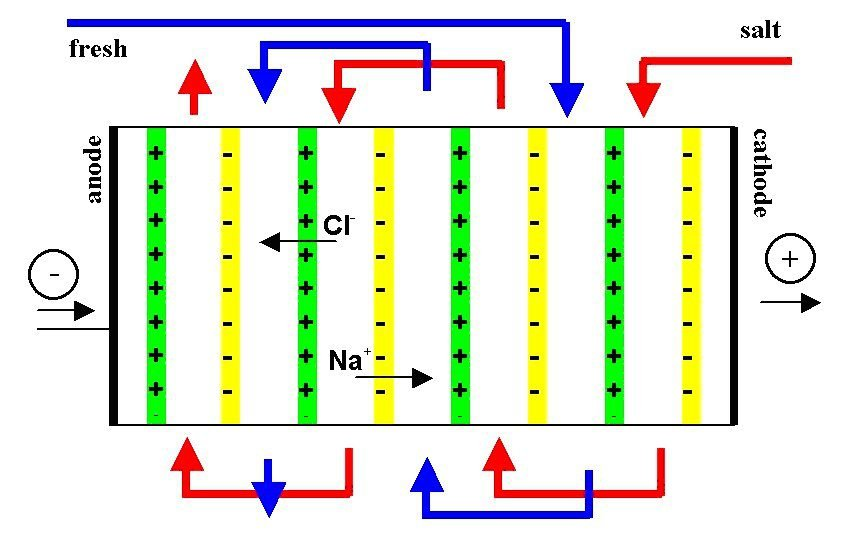This is a reverse electrodialysis cell.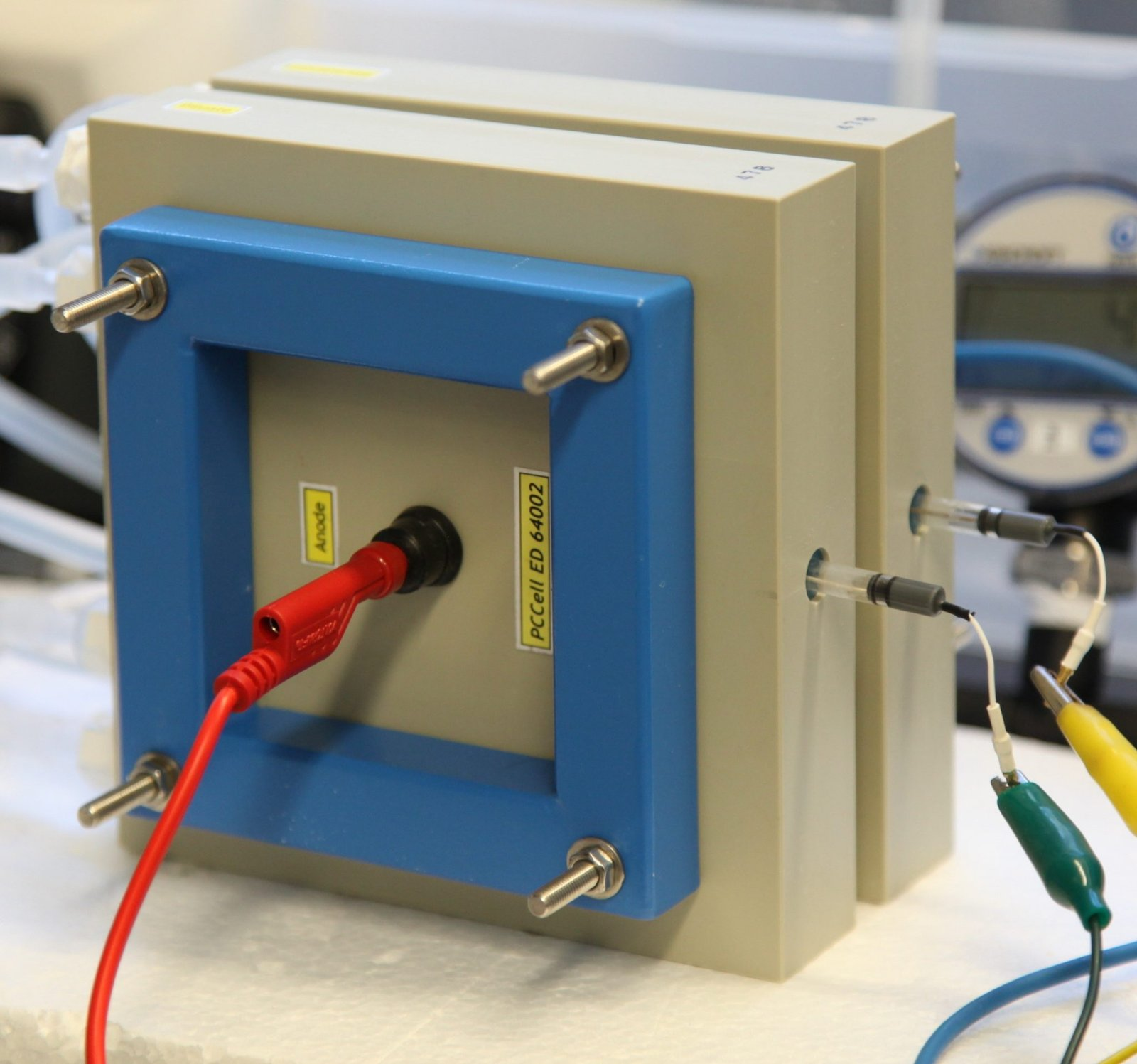The positive charged membrane allows the passage of negative ions and vice-versa. This technology is still in research phase and many membrane materials with nanometric thickness are being studied.